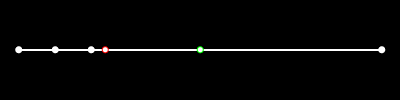# Crv Deviation Bug

Hey @dale,

Thanks for yesterday, and here’s that GetDistancesBetweenCurves bug. It’s in the repo I shared with you, along with sample geometry.

``````if (Curve.GetDistancesBetweenCurves(crv0, crv1, 0.001,
out max, out maxT0, out maxT1,
out min, out minT0, out minT1))
{
var d0 = crv0.Domain.T1 - crv0.Domain.T0;
var d1 = crv1.Domain.T1 - crv0.Domain.T0;

var t0 = crv0.Domain.NormalizedParameterAt(maxT0);
var t1 = crv1.Domain.NormalizedParameterAt(maxT1);
var pt0 = crv0.PointAtNormalizedLength(t0);
var pt1 = crv1.PointAtNormalizedLength(t1);

RhinoApp.WriteLine("Max: {0:N3}, MaxT0: {1:N3}, MaxT1: {2:N3}.", max, maxT0, maxT1);
RhinoApp.WriteLine("t Delta - Crv0: {0:N3}, Crv0: {1:N3}", d0, d1);
RhinoApp.WriteLine("Normalized t - Crv0: {0:N3}, Crv1: {1:N3}", t0, t1);

var line = new Line(pt0, pt1);
RhinoApp.WriteLine("Distance from Pts at Normalized t's: {0:N3}", line.Length);
``````
``````Command: CrvDeviationBug
Max: 5.123, MaxT0: 3.672, MaxT1: 0.493.
Domain - Crv0: 6.000, Crv0: 1.000
Normalized t - Crv0: 0.612, Crv1: 0.493
Distance from Pts at Normalized t's: 3.245
``````

The bug is on `maxT0`. The `max` distance is likely precise, but `maxT0` should be slightly less than 3.0 (`t0 ~ 0.49`).

Hi @EricM,

Using your sample curves, this seems to produce the same results as the `CrvDeviation` command.

``````protected override Result RunCommand(RhinoDoc doc, RunMode mode)
{
var go = new GetObject { GeometryFilter = ObjectType.Curve };
go.SetCommandPrompt("Select curves to test");
go.GetMultiple(2, 2);
if (go.CommandResult() != Result.Success)
return go.CommandResult();

var curveA = go.Object(0).Curve();
var curveB = go.Object(1).Curve();
if (null == curveA || null == curveB)
return Result.Failure;

var rc = Curve.GetDistancesBetweenCurves(
curveA,
curveB,
doc.ModelAbsoluteTolerance,
out var maxDistance,
out var maxDistanceParameterA,
out var maxDistanceParameterB,
out var minDistance,
out _,
out _
);

if (rc)
{
var line = new Line
{
From = curveA.PointAt(maxDistanceParameterA),
To = curveB.PointAt(maxDistanceParameterB)
};

RhinoApp.WriteLine("Minimum deviation = {0}", minDistance);
RhinoApp.WriteLine("Maximum deviation = {0}", maxDistance);
}

return Result.Success;
}
``````

– Dale

Thanks @dale, that does work.

The difference is normalizing the pt in the domain vs. using the domain value. This is something I need to learn. Maybe @rajaa can point me somewhere to gain a better understanding?

I looked at the knot vector of the curve I thought had a bugged normalized t and saw that it was non-uniform. But _MakeUniform improved the result only slightly. So knot vectors have a sort of “amplitude”? And that amplitude differs from single and multi-span curves even though the knot vectors are uniform?

``````  Rhino object: curve
DEVELOPER DEBUGGING INFORMATION ONLY
Use the Rhino "What" command.
Runtime serial number: 205
name: ""
id: F8D4BFBB-C324-42b9-B9E6-D362BF066726
layer index: 0
render material index: -1 (from layer)
ON_NurbsCurve dim = 3 is_rat = 0
order = 4 cv_count = 6
Knot Vector ( 8 knots )
index                     value  mult       delta
0                        0     3
3                      2.5     1         2.5
4                        5     1         2.5
5                        6     3           1
Control Points  6 non-rational points
index               value
CV[ 0] (-16.28236526962759, -8.1453974912651859, 0)
CV[ 1] (-4.0601557822207042, -5.2251225768485838, 0)
CV[ 2] (-4.0601557822207042, 7.5719088468288831, 0)
CV[ 3] (-1.7911892111280423, 13.036955444990724, 0)
CV[ 4] (9.6458013429528933, -5.6414567479583795, 0)
CV[ 5] (18.028254589927116, -4.9149422797722622, 0)
``````

The “parameter” for PointAtNormalizedLength is relative to the curve’s actual length, not its (parameter space) domain.

For example, for this cubic Bezier:• The white points are control points.
• The red point is at the 0.5 parameter of a [0.0, 1.0] interval.
• The green point was returned from PointAtNormalizedLength(0.5).